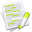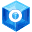#wx.richtext.RichTextRange¶

This stores beginning and end positions for a range of data.

##Class Hierarchy¶Inheritance diagram for class RichTextRange:

##Methods Summary¶

 __init__ Default constructor. Contains Returns True if pos was within the range. FromInternal Converts the internal range, which uses the first and last character positions of the range, to the API-standard range, whose end is one past the last character in the range. Get Return the start and end properties as a tuple. GetEnd Gets the end position. GetIM Returns an immutable representation of the wx.RichTextRange object, based on namedtuple. GetLength Gets the length of the range. GetStart Returns the start position. IsOutside Returns True if this range is completely outside range. IsWithin Returns True if this range is completely within range. LimitTo Limit this range to be within range. SetEnd Sets the end position. SetRange Sets the range start and end positions. SetStart Sets the start position. Swap Swaps the start and end. ToInternal Converts the API-standard range, whose end is one past the last character in the range, to the internal form, which uses the first and last character positions of the range. __bool__ __getitem__ __len__ __nonzero__ __reduce__ __repr__ __setitem__ __str__ __ne__ Inequality operator. __add__ Adds a range to this range. __sub__ Subtracts a range from this range. __eq__ Equality operator.

##Class API¶

class wx.richtext.RichTextRange(object)

Possible constructors:

RichTextRange()

RichTextRange(start, end)

RichTextRange(range)


This stores beginning and end positions for a range of data.

### Methods¶

__init__(self, *args, **kw)Overloaded Implementations:

__init__ (self)

Default constructor.

__init__ (self, start, end)

Constructor taking start and end positions.

Parameters
• start (long) –

• end (long) –

__init__ (self, range)

Copy constructor.

Parameters

range (wx.richtext.RichTextRange) –

Contains(self, pos)

Returns True if pos was within the range.

Does not match if the range is empty.

Parameters

pos (long) –

Return type

bool

FromInternal(self)

Converts the internal range, which uses the first and last character positions of the range, to the API-standard range, whose end is one past the last character in the range.

In other words, one is added to the end position. (n, n+1) is the range of a single character.

Return type

wx.richtext.RichTextRange

Get(self)

Return the start and end properties as a tuple.

Return type

tuple

Returns

( start, end )

GetEnd(self)

Gets the end position.

Return type

long

GetIM(self)

Returns an immutable representation of the wx.RichTextRange object, based on namedtuple.

This new object is hashable and can be used as a dictionary key, be added to sets, etc. It can be converted back into a real wx.RichTextRange with a simple statement like this: obj = wx.RichTextRange(imObj).

GetLength(self)

Gets the length of the range.

Return type

long

GetStart(self)

Returns the start position.

Return type

long

IsOutside(self, range)

Returns True if this range is completely outside range.

Parameters

range (wx.richtext.RichTextRange) –

Return type

bool

IsWithin(self, range)

Returns True if this range is completely within range.

Parameters

range (wx.richtext.RichTextRange) –

Return type

bool

LimitTo(self, range)

Limit this range to be within range.

Parameters

range (wx.richtext.RichTextRange) –

Return type

bool

SetEnd(self, end)

Sets the end position.

Parameters

end (long) –

SetRange(self, start, end)

Sets the range start and end positions.

Parameters
• start (long) –

• end (long) –

SetStart(self, start)

Sets the start position.

Parameters

start (long) –

Swap(self)

Swaps the start and end.

ToInternal(self)

Converts the API-standard range, whose end is one past the last character in the range, to the internal form, which uses the first and last character positions of the range.

In other words, one is subtracted from the end position. (n, n) is the range of a single character.

Return type

wx.richtext.RichTextRange

__bool__(self)

__getitem__(self, idx)

__len__(self)

__nonzero__(self)

__reduce__(self)

__repr__(self)

__setitem__(self, idx, val)

__str__(self)

__ne__(self)

Inequality operator.

Parameters

range (wx.richtext.RichTextRange) –

__add__(self)

Adds a range to this range.

Parameters

range (wx.richtext.RichTextRange) –

__sub__(self)

Subtracts a range from this range.

Parameters

range (wx.richtext.RichTextRange) –

__eq__(self)

Equality operator.

Returns True if range is the same as this range.

Parameters

range (wx.richtext.RichTextRange) –

### Properties¶

End

Length

Start